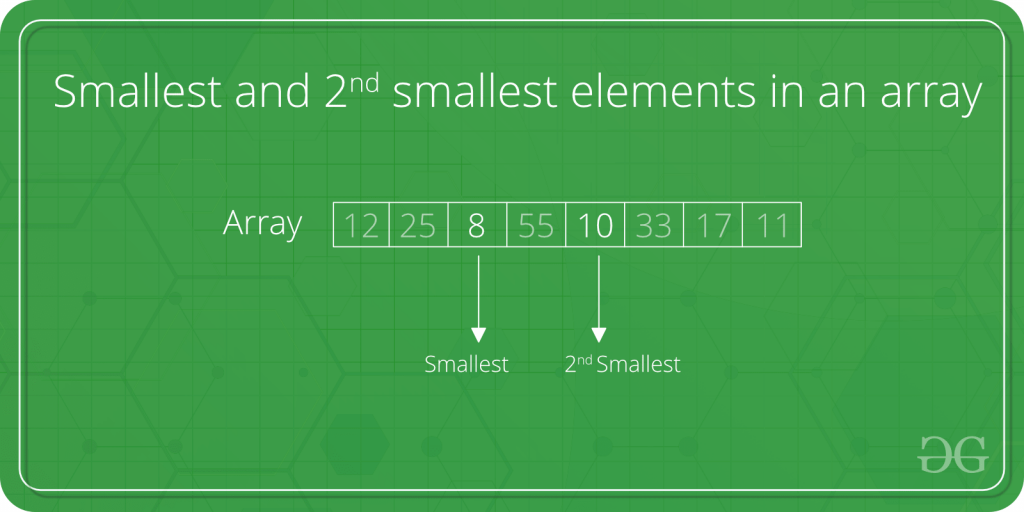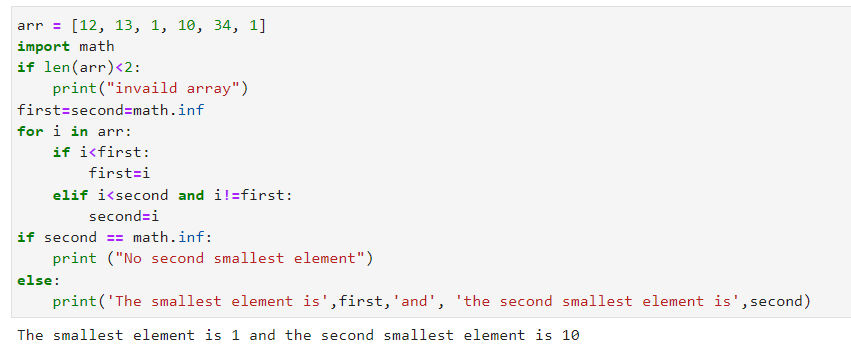# 每日一题【20220411】

### 题目

Find the smallest and second smallest elements in an array(查找数组中第二小的元素)

Difficulty Level : Basic

Find the smallest and second smallest elements in an array.Example:

### 解题思路

A Simple Solution is to sort the array in increasing order. The first two elements in sorted array would be two smallest elements. Time complexity of this solution is O(n Log n).
A Better Solution is to scan the array twice. In first traversal find the minimum element. Let this element be x. In second traversal, find the smallest element greater than x. Time complexity of this solution is O(n).
The above solution requires two traversals of input array.
An Efficient Solution can find the minimum two elements in one traversal. Below is complete algorithm.
Algorithm:

Implementation:

Output :

The same approach can be used to find the largest and second largest elements in an array.
Time Complexity: O(n)

• 稳健!